# Determination of quantity supplied by firm in perfectly competitive market in the long run

This article describes the process by which a firm selling a good, service or commodity in a perfectly competitive market decides the quantity to be supplied.

The chief characteristic of a perfectly competitive market is that:

1. Each firm is small in size relative to the totality of all firms supplying the commodity, and is competing solely on price (i.e., with no product differentiation).
2. The firm is a price taker rather than a price setter, i.e., it has no leeway in deciding the price and must stick to the price prevailing in the market.
3. Whatever quantity the firm produces within its natural limitations can be completely sold at the market, i.e., the firm does not have to worry about unsold inventory as long as it prices products at the market price.

Setting the price at the market price is not (necessarily) enforced through any legal regulation, but is forced for the following two reasons:

• Because the firm is very small relative to the whole market, reducing the price below the market price will shift much more consumer demand to that firm than the firm can handle. Thus, the firm does not reduce demand significantly below the market price.
• Similarly, increasing the price above the market price will cause the firm to lose all its customers to competing firms.

## Cost and revenue definitions

We assume that the firm has two costs:

• Fixed costs are fixed in advance and cannot be altered in the short run. These may include investments in land or other fixed assets that cannot be acquired or got rid of quickly.
• Variable costs are costs that vary with the quantity produced.

We define:

Cost notion Acronym Definition How does it vary with quantity?
Fixed cost FC Cost of expenditure on fixed factors of production that cannot be varied in the short run Independent of quantity
Variable cost VC Cost of expenditure on factors of production that can be varied in the short run Increases with quantity
Total cost TC Fixed cost + Variable cost Increases with quantity
Average cost ATC, AC (Total cost)/Quantity Generally, first decreases and then increases with quantity
Average fixed cost AFC (Fixed cost)/Quantity Decreases with quantity (by definition, because denominator is increasing and numerator is constant).
Average variable cost AVC (Variable cost)/Quantity Generally, increases with quantity when quantity is large enough. For small quantities, it may decrease with quantity.
Marginal cost MC Derivative of total cost with respect to quantity; since fixed cost is independent of quantity, this is the same as the derivative of variable cost with respect to quantity Generally, increases with quantity when quantity is large enough. For small quantities, it may decrease with quantity.
Market price P The unit price in the market. Not under the control of the firm. Independent of quantity
Revenue R Revenue earned by producing a given quantity of the commodity. It equals the product of quantity produced and the (unit) market price. Note that because of the small size of the firm relative to the market, it must sell everything it produces at the market price Increases with quantity (by definition).
Marginal revenue MR Equals the market price P Independent of quantity
Profit (not accounting for fixed costs)  ? Equals R - VC, same as Q(P - AVC) where Q is the quantity produced, P is the unit price, and AVC is the average variable cost at the given value of Q. This is what the firm tries to maximize in the short run, and the firm chooses to be idle if there is no choice of production quantity at which this number is positive. Maximum at some particular choice of quantity (see short-run choice)
Profit (accounting for fixed costs)  ? Equals R - TC, same as Q(P - ATC), where Q is the quantity produced, P is the unit price, and AC is the average (total of fixed and variable) cost. In the short run, the firm may choose to produce even if the profit, after accounting for fixed costs, is negative. In the long run, when the firm can choose fixed costs, the firm will choose to produce only if the profit is positive. Maximum for some particular choices (see long-run choice)

## Construction of the long run cost functions and curves based on short run data

In the long run, the firm can choose both its fixed and its variable costs. Much of the long run analysis is similar to the short run analysis, with the main qualitative difference being that we are interested in total cost and average total cost instead of variable cost and average variable cost. Moreover, the fixed costs are now variable, which means that the choice of fixed costs can be changed based on the quantity that is expected to be made. A key difference is that the average total cost curve and marginal cost curve do not begin together at quantity zero, unlike the case of the AVC and MC curves. Rather, the ATC curve begins higher than the MC curve. This reflects the fact that even to produce a very small quantity, some positive fixed costs are necessary.

### Note on the set of fixed cost scenarios

We will use the term fixed cost scenario for a particular choice of allocation of fixed costs. For each fixed cost scenario, we have curves for the total cost, average total cost, variable cost, average variable cost, and marginal cost, all plotting them as functions of quantity.

Note that a given fixed cost scenario includes a numerical value of fixed cost, but may not be completely described by it: there may be many different fixed cost scenarios that involve the same numerical quantity of fixed cost allocated in different ways.

### Typical nature of trade-off: higher initial fixed cost enables greater minimum efficient scale and slower-growing marginal cost

As a general rule, the higher the initial fixed cost, the slower the growth of marginal cost, the larger the minimum efficient scale, and the larger the maximum production capacity. This is not universally true, and in particular, higher fixed costs may also be associated with higher marginal costs in some cases.

• How higher fixed costs can lead to greater production capacity: Consider a scenario where investments in factory floor space and machines are treated as fixed costs, whereas person-hours to operate the machines are variable costs. In this case, higher fixed costs means a larger factory and more machines, and therefore, more throughput at full capacity.
• How higher fixed costs can lead to greater minimum efficient scale: Consider the same scenario as for the preceding point. The minimum efficient scale in this case is likely achieved when the number of people operating the factory is at the level the factory can comfortably accommodate (so that people can help each other, but not get in each other's way) and for a typical number of work-hours (rather than having to do overtime). The larger the factory, the larger this scale. Beyond this scale, average variable costs start rising because the factory gets more crowded, or people need to be paid overtime.

### Construction of the total cost curve for long run choice

For each fixed cost scenario, we can construct a total cost curve specific to that value of fixed cost. This total cost curve is obtained by shifting the variable cost curve (for that particular choice of fixed cost) upward by the fixed cost value.

When making a long run choice, we can, for any given quantity to be produced, compare the total cost values for all the total cost curves (that can be achieved by choosing different fixed costs). We pick the lowest of these values, i.e., for a given quantity, we pick the minimum of the total cost values for all the short-run total cost curves possible.

We do the above for every choice of quantity to be produced. We then join all these minimum points to get the long-run total cost curve.

In other words, the long-run total cost function is the minimum of all the short-run total cost functions. Geometrically, the long-run total cost curve is obtained as the highest curve that bounds from below all the short-run total cost curve.

### Construction of the average total cost curve for long-run choice

This proceeds based on the average total cost curves for each short-run choice in the same way as the construction of the total cost curve does. The following caveat is worth keeping in mind, though: each of the average total cost curves for short-run choice approach infinity as the quantity produced approaches zero (unless there are zero fixed costs). This might result in the average total cost curve for long-run choice also having a discontinuity at quantity zero. This is not a problem per se.

### Relation with minimum efficient scale

The choice of fixed cost scenario that minimizes total cost (or equivalently, minimizes average total cost) for a given quantity of production is such that the given quantity of production is often close to the minimum efficient scale for that choice of fixed cost scenario. The intuition is that in most cases, if the quantity of production is known beforehand, it makes sense to shift costs away from the rising part of the AVC curve and push them onto fixed costs. In words, if it's known that a certain amount of material needs to be produced, it makes the most sense to build a factory that can produce that much under normal conditions. Investing in too big a factory means that you wasted fixed costs on production capacity that won't get used. Investing in too small a factory means that producing the required amount means you have to overuse some resources in a costly manner (such as paying workers for overtime or overcrowding the factory).

### Construction of the long-run marginal cost curve

The long-run marginal cost function is the derivative of the long-run total cost function. Note, however, that the long-run total cost function is the minimum of all the short-run total cost functions, and the short-run marginal cost functions (the critical components in short-run optimization) are obtained by differentiating these short-run total cost functions. There is no straightforward way of expressing the relationship between the long-run marginal cost curve and the short-run marginal cost curves.

## Qualitative nature of the long-run marginal cost curve

### Case of flat long-run marginal cost curve

Further information: constant cost industry

A flat long-run marginal cost curve, i.e., a situation where the long-run marginal cost is constant, means that production enjoys constant returns to scale. Consider, for instance, a situation where the output of a factory is a Cobb-Douglas production function of the form: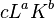$cL^aK^b$

and the expenditure is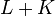$L + K$. Here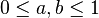$0 \le a,b \le 1$ and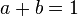$a + b = 1$. For any given quantity of target output$Q$, we would choose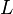$L$ and$K$ in the ratio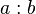$a:b$ so as to produce that output. Specifically, for cost$C$,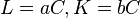$L = aC, K = bC$ so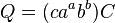$Q = (ca^ab^b)C$. In other words, the total output is proportional to the cost. Or equivalently, the cost needed to produce a given output is proportional to the output. Note, however, that this is true only in the long run, when we can choose$L$ and$K$ arbitrarily.

For convenience, assume that$K$ is the fixed cost in the short run and$L$ is the variable cost. Then, the variable cost needed to produce output$Q$ is$(Q/cK^b)^{1/a}$ and the average variable cost is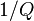$1/Q$ times that quantity. The marginal cost is$(1/a)(1/cK^b)^{1/a}Q^{1/a - 1}$. This is a positive and increasing function of$Q$, so the marginal cost curve is upward-sloping. The upshot:

• The long-run marginal cost curve is flat.
• The short-run marginal cost curve is upward-sloping.

Constant cost industries are also said to have constant returns to scale.

### Case of upward-sloping long-run marginal cost curve

Further information: increasing cost industry

This can occur in situations where we don't have much flexibility with respect to fixed costs. It can also describe situations where there are strict limits on available resources. Industries with upward-sloping marginal cost curves are termed increasing cost industries. Increasing cost industries are also said to have decreasing returns to scale.

### Case of downward-sloping long-run marginal cost curve

Further information: decreasing cost industry

This can occur in situations where some parts of the fixed costs scale sub-linearly with the amount to be produced. For instance, setup costs for factories may not double when the factory size doubles. Decreasing cost industries are also said to have increasing returns to scale.

## Calculation problem for the firm

This calculation problem assumes that the firm has a long-run expected price known to it, and attempts to optimize for that expected price. Long-run calculation facing a probability distribution for a price, or facing seasonal variation in the price, becomes trickier and is not discussed here.

The calculation problem is very similar to the calculation problem in the determination of quantity supplied by firm in perfectly competitive market in the short run.

• The long-run marginal cost curve of the firm.
• The long-run expected market price facing the firm.

### Goal of the firm

The firm's goal is to use the information available to it (its long-run marginal cost curve and the long-run expected market price) to choose a quantity to produce so as to maximize its profit (note that fixed costs are treated as sunk costs and thus do not affect the profit calculation). If the firm chooses to product a quantity$Q$, the profit (the objective function to be maximized)) is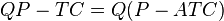$QP - TC = Q(P - ATC)$ where$TC$ is the total cost and$ATC$ is the average total cost, both themselves functions of$Q$.

### Construction of the long-run supply curve

The long run supply curve is constructed as follows: for each possible value of market price$P$, the firm solves the optimization problem above and computes the quantity to produce. This quantity to produce is then plotted as a function of market price, with price on the vertical axis and quantity on the horizontal axis.

It will turn out that the following hold:

• The long run supply curve coincides with an upward sloping part of the long run marginal cost curve, and the formal mathematical analysis is similar to that for the short run, with the only difference being that we are now considering long-run marginal cost instead of short-run marginal cost.
• For an increasing cost industry, the long-run supply curve coincides with the long-run marginal cost curve.
• For a constant cost industry or decreasing cost industry, the firm simply produces at its maximum capacity as long as the average total cost at maximum capacity is less than or equal to the price, otherwise the industry is not feasible. In other words, the average total cost at maximum capacity is the reservation price.